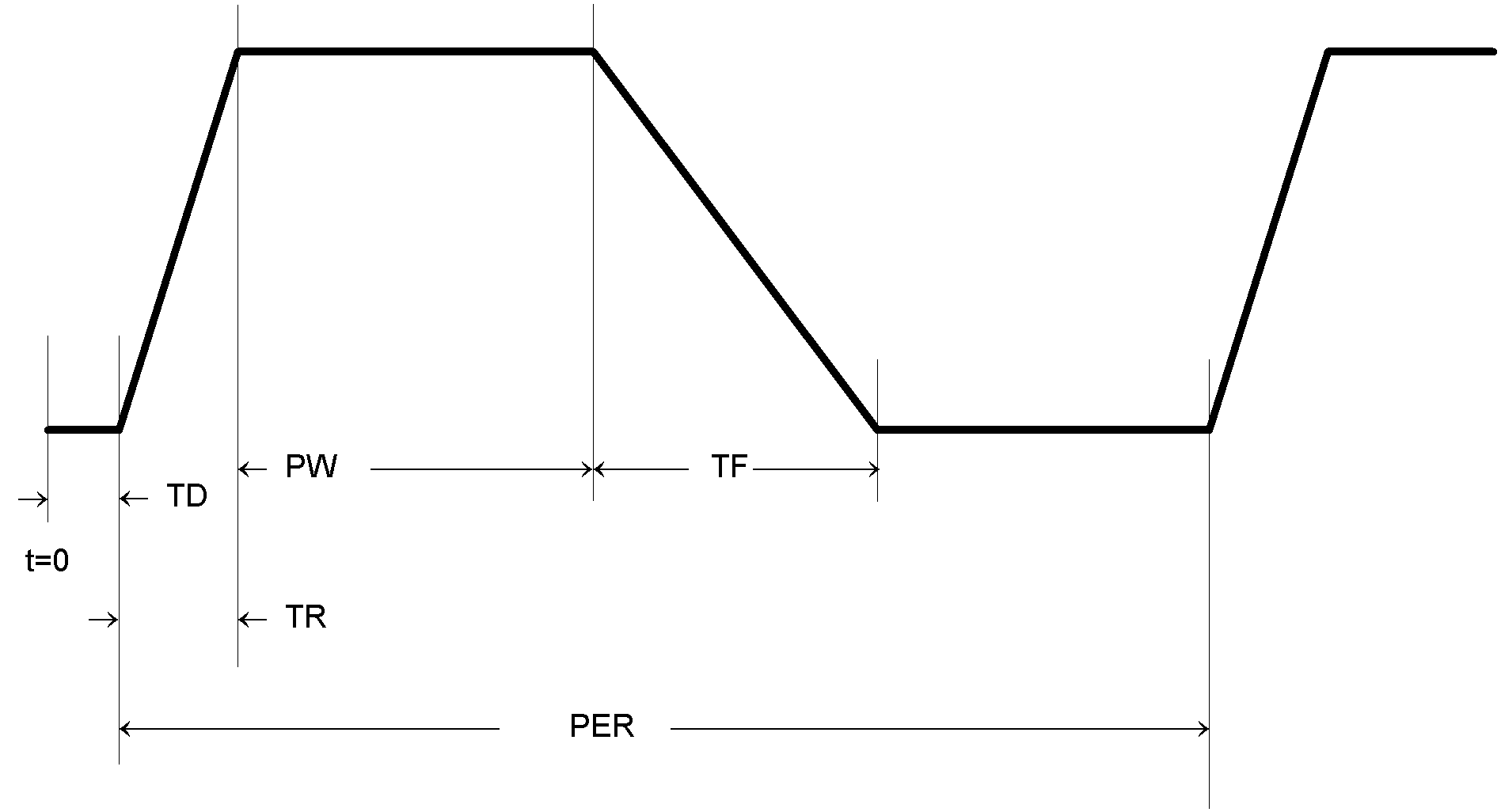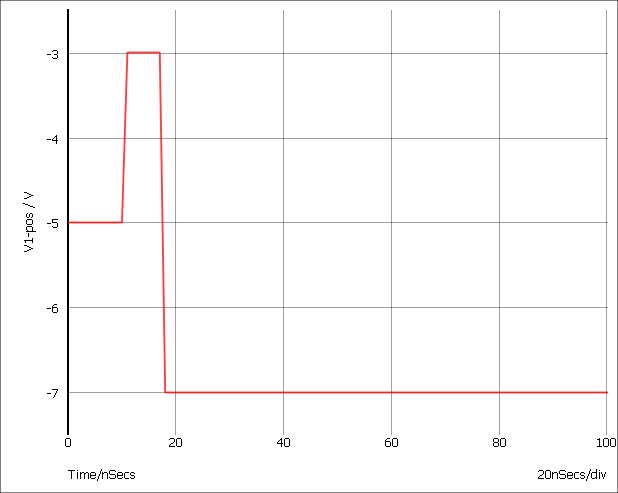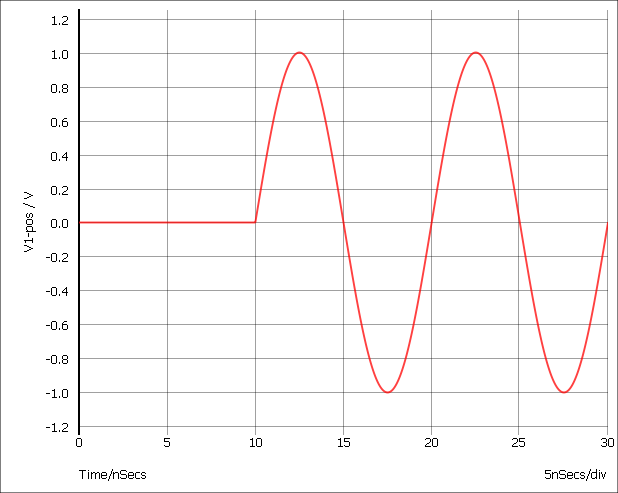# Voltage Source

In this topic:

## Netlist Entry

Vxxxx n+ n- [[DC] dcvalue] [DCOP] [INFCAP] [AC magnitude [phase]]
[transient_spec]
 N+ Positive node N- Negative node DCOP If this is specified, the voltage source will only be active during the DC operating point solution. In other analyses, it will behave like an open circuit. This is an effective method of creating a 'hard' initial condition. See Alternative Initial Condition Implementations for an example. INFCAP If specified, the voltage source will behave as an infinite capacitor. During the DC operating point solution it will behave like an open circuit. In the subsequent analysis, it will behave like a voltage source with a value equal to the solution found during the operating point. Note that the device is inactive for DC sweeps - as all capacitors are. dcvalue Value of source for dc operating point analysis magnitude AC magnitude for AC sweep analysis. phase phase for AC sweep analysis transient_spec Specification for time varying source as described in the following table.
 Type Description PULSE Pulse source. Also generates, ramps, sawtooths and triangles PWL Piece wise linear source. Can create any waveform PWLFILE As PWL but get definition from a file SIN Sine wave EXP Exponential signal SFFM Single frequency FM NOISE Real time noise source

## Pulse Source

PULSE ( v1 v2 [td [tr [tf [pw [per ]]]]] )

Where:

 Name Description Default v1 Initial value (V,A) Compulsory v2 Pulsed value (V,A) Compulsory td Delay time (S) Default if omitted = 0 tr Rise time (S) Default if omitted, negative or zero = Time stepa tf Fall time (S) Default if omitted, negative or zero = Time step pw Pulse width (S) Default if omitted or negative = Stop timeb per Period (S) Default if omitted, negative or zero = Stop time
 a. Time step is set up by the .TRAN simulator statement which defines a transient analysis. Refer to .TRAN. b. Stop time refers to the end time of the transient analysis.

SIMetrix deviates from standard SPICE in the action taken for a pulse width of zero. Standard SPICE treats a zero pulse width as if it had been omitted and changes it to the stop time. In SIMetrix a zero pulse width means just that.

Both the above examples give a pulse lasting 5$\mu$S with a period of 10$\mu$S, rise and fall times of 100nS and a delay of 0. The voltage source has a 0V base line and a pulse of 5V while the current source has a 0mA base line and a pulse of 1mA.### Examples## Piece-Wise Linear Source

PWL ( t1 v1 [t2 v2 [t3 v3 [... ]]] )

Each pair of values (ti vi) specifies that the value of the source is vi at time = ti. The value of the source at intermediate values of time is determined by using linear interpolation on the input values.

Although the example given below is for a voltage source, the PWL stimulus may be used for current sources as well.

### ExampleGives:## PWL File Source

PWLFILE filename

This performs the same function as the normal piece wise linear source except that the values are read from a file named filename.

The file contains a list of time voltage pairs in text form separated by any whitespace character (space, tab, new line). It is not necessary to add the '+' continuation character for new lines but they will be ignored if they are included. Any non-numeric data contained in the file will also be ignored.

### Notes

The PWLFILE source is considerably more efficient at reading large PWL definitions than the standard PWL source. Consequently it is recommended that all PWL definitions with more than 200 points are defined in this way.

The data output by Show /file is directly compatible with the PWLFILE source making it possible to save the output of one simulation and use it as a stimulus for another. It is recommended, however, that the results are first interpolated to evenly spaced points using the Interp() function.

The use of engineering suffixes (e.g. k, m, p etc.) is not supported by PWLFILE.

The PWLFILE source is a feature of SIMetrix and does not form part of standard SPICE.

Note, you can use the simulator statements .FILE and .ENDF to define the contents of the file. E.g.

Vpwl1 N1 N2 PWLFILE pwlSource
...
.FILE pwlSource
...
...
.ENDF

This will be read in much more efficiently than the standard PWL and is recommended for large definitions. See .FILE and .ENDF.

## Sinusoidal Source

SIN[E] ( vo va [freq [delay [theta [ phase]]]] )

Where:

 Name Description Default vo Offset (V,A) Compulsory va Peak (V,A) Compulsory freq Frequency (Hz) Default if omitted or zero= 1/Stop timea delay Delay (seconds) Default if omitted = 0 theta Damping factor (1/seconds) Default if omitted = 0 phase Phase in degrees Default if omitted = 0
 a. Stop time refers to the end time of the transient analysis.

The shape of the waveform is described by:

 0 to delay: vo delay to Stop time $vo + va \cdot \text{e}^{-(t-\textit{delay})..\textit{theta}} \cdot \sin(2\pi(\textit{freq} \cdot (t - \textit{delay}) + \textit{phase}/360))$

### ExampleGives output of:## Exponential Source

EXP ( v1 v2 [td1 [tau1 [td2 [tau2 ]]]] )

Where:

 Name Description Default v1 Initial value (V,A) Compulsory v2 Pulsed value (V,A) Compulsory td1 Rise delay time Default if omitted or zero: 0 tau1 Rise time constant Default if omitted or zero: Time stepa td2 Fall delay time Default if omitted or zero: td1 + Time step tau2 Fall time constant Default if omitted or zero: Time step
 a. Time step is set up by the .TRAN simulator directive which defines a transient analysis. Refer to .TRAN.
Defined by:
 0 to td1: $v1$ td1 to td2: $v1 + (v2 - v1). [ 1 - \text{e}^{-(t-td1)/tau1}]$ td2 to stop time: $v1 + (v2 - v1). [ 1 - \text{e}^{-(t-td1)/tau1}] + (v2 - v1). [ 1 - \text{e}^{-(t-td2)/tau2}]$

## Single Frequency FM

SFFM ( vo va [fc [mdi [fs ]]] )

Where:

 Name Description Default vo Offset (V,A) Compulsory va Amplitude (V,A) Compulsory fc Carrier frequency (Hz) Default if omitted or zero = 1/Stop timea mdi Modulation index Default if omitted = 0 fs Signal frequency (Hz) Default if omitted or zero = 1/Stop time
 a. Stop time refers to the end time of the transient analysis.

Defined by: $vo + va\cdot\sin[2\pi\cdot \textit{fc}\cdot t + \textit{mdi}\cdot\sin(2\pi\cdot\textit{fs}\cdot t)]$

## Noise Source

noise interval rms_value [start_time [stop_time]]

Source generates a random value at interval with distribution such that spectrum of signal generated is approximately flat up to frequency equal to 1/(2*interval). Amplitude of noise is rms_value volts. start_time and stop_time provide a means of specifying a time window over which the source is enabled. Outside this time window, the source will be zero. If stop_time is omitted or zero a value of infinity will be assumed.

## Extended PWL Source

PWLS [TIME_SCALE_FACTOR=time_factor] [VALUE_SCALE_FACTOR=value_factor]
pwls_spec [ pwls_spec ... ]
Where:
time_factor Scales all time values in definition by time_factor
value_factor Scales all magnitude values by value_factor
pwls_spec may be one of the following:
 (time, value) Creates a single data point. time is relative to the current context. (+time, value) Creates a single data point. time is relative to the previous point. REPEAT FOR n pwls_spec ENDREPEAT Repeats pwls_spec n times. REPEAT FOREVER pwls_spec ENDREPEAT Repeats pwls_spec forever SIN sine_parameters END Creates a sinusoid. See table below for definition of sine_parameters PULSE pulse_parameters END Creates a pulse train. See table below for definition of pulse_parameters FILE file_name Loads a definition from file file_name. If the name contains spaces, it must be quoted WAV wav_file_parameters END Loads a WAV file

### Sine Parameters

 Name Description Default Compulsory FREQ Frequency N/A Yes PEAK Peak value of sine 1.0 No OFFSET Offset 0.0 No DELAY Delay before sine starts. 0.0 No PHASE Phase 0.0 No CYCLES Number of cycles. Use -1.0 for infinity -1.0 No MINPOINTS Minimum number of timesteps used per cycle 13 No RAMP Frequency ramp factor 0.0 No
The sine value is defined as follows:
if t>0 OR DELAY<0
PEAK * SIN(f*2pi*t+PHASE*pi/180) + OFFSET
else
PEAK * SIN(PHASE*pi/180) + OFFSET
Where:
• f = FREQ + t$\times$RAMP
• t = time - tref - DELAY
• time is the global simulation time
• tref is the reference time for this spec

### Pulse Parameters

 Name Description Default Compulsory V0 Offset 0 No V1 Positive pulse value 1.0 No V2 Negative pules value -1.0 No RISE Rise time i.e time to change from V2 to V1 PERIOD/1000 No FALL Fall time i.e time to change from V1 to V2 PERIOD/1000 No WIDTH Positive pulse width (PERIOD-RISE-FALL)/2 No PERIOD Period N/A Yes DELAY Delay before start 0 No CYCLES Number of complete cycles. -1 means infinity -1 No
RISE, FALL, WIDTH and PERIOD must be greater than zero. DELAY must be greater than or equal to zero

### WAV Parameters

 Name Description Default Compulsory FILE WAV File path N/A Yes PEAK Peak value 1.0 No INTERVAL Time duration of signal in seconds Whole signal No CHANNEL Data Channel. 0 or 1 - currently only 1 or 2 channels is supported. Can also use 'L', 'R', 'LEFT' and 'RIGHT' 0 No SAMPLE_RATE Sample rate Read from file No

Reads a WAV format data file. WAV is a format used generally for sampled audio data. SIMetrix can read these files subject to these conditions:

• No more than 2 channels
• Bits per sample is 8, 16, 24 or 32
• Data is uncompressed